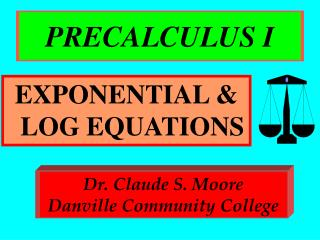DownloadDownload PresentationPRECALCULUS I

# PRECALCULUS I

Télécharger la présentation## PRECALCULUS I

- - - - - - - - - - - - - - - - - - - - - - - - - - - E N D - - - - - - - - - - - - - - - - - - - - - - - - - - -
##### Presentation Transcript

1. PRECALCULUS I EXPONENTIAL & LOG EQUATIONS Dr. Claude S. MooreDanville Community College

2. EXPONENTIAL & LOG INVERSE PROPERTIES 1. loga ax= x ln ex= x . 2. a log a x = x e lnx = x .

3. ONE-TO-ONE PROPERTIES 1. loga x = loga y iff x = y. 2. a x= a y iff x = y. 3. a x= b x iff a = b.

4. TO SOLVE ... • Exponential equation: Isolate exponential expression; take log of both sides and solve. • Logarithm equation: Rewrite as exponential equation and solve.

5. EXAMPLE 1 • Solve (no calculator): 3x-1 = 243 3x-1 = 35 • Thus, x - 1 = 5 or x = 6.

6. EXAMPLE 2 • Solve (3-decimal places): 4e2x = 50e2x = 50/4 = 12.5 • ln e2x = 2x(ln e) = ln 12.5 • x = (ln 12.5)/2 = 1.263

7. EXAMPLE 3 Solve (3-decimal places):ln (x-2) + ln (2x+3) = ln x2 ln (x-2)(2x+3) = ln x2 ln (2x2-x-6) = ln x2 2x2-x-6 = x2

8. EXAMPLE 3 continued 2x2-x-6 = x2 x2-x-6 = 0 (x-3)(x+2) = 0 x-3 = 0 or x+2 = 0 x = 3 or x = -2

9. EXAMPLE 3 concluded ln (x-2) + ln (2x+3) = ln x2 Domain x-2>0, yields x>2 2x+3 > 0, yields x > -3/2 x2 > 0, yields x  0. So answer is x = 3.

10. STUDY AND WORK HARD... ...the sky is the limit!